# What is Indifference Curve ?

• Last Updated : 23 May, 2022

One cannot put a numerical value on the level of satisfaction gained from the consumption of goods. However, they can tell their preference between two goods, i.e., which good gives them more or less satisfaction. This satisfaction level is depicted through an indifference curve. Therefore, a curve or a graphical representation of the combination of different goods providing the same satisfaction level to the consumer is known as Indifference Curve.
As all the combinations provide the consumer with an equal level of satisfaction, they prefer the goods equally. In other words, at any point of the indifference curve gives the same satisfaction level to the consumer. The same satisfaction level gained by the different combinations of two goods makes the consumer indifferent; hence, the name indifference curve. Because of this reason, an individual can use the indifference curve to depict the demand pattern and preferences of a consumer for a different set of commodities.

### Indifference Curve Analysis

A process of analyzing a simple two-dimensional graph representing two goods, one on the x-axis and the other on the y-axis is known as an Indifference Curve Analysis. If the graph of the combination of goods is on the line or curve, it means that the consumer gains the same satisfaction level or utility from the goods and thus, does not have any preference for the goods. For example, a child may gain the same satisfaction level from one ice cream and two chocolates, or three ice creams and one chocolate.

### Indifference Map

When more than one curve is represented on a graph showing a different combinations of two different goods on each curve, it is known as an Indifference Map. Each indifference curve on that graph shows one satisfaction level all along the curve. In other words, the representation of consumer preferences by a number of indifference curves is known as an indifference map. An indifference map represents every possible indifference curve that the consumer has, which helps in ranking their preferences. Also, the combination of goods on the higher indifference curve gives a higher satisfaction level to the consumer. Therefore, the highest of the indifference curves of an indifference map is preferred by a consumer.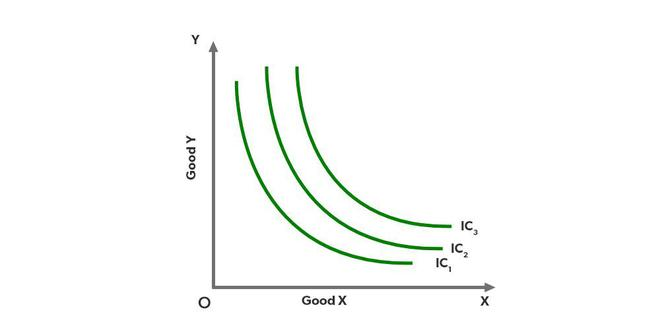Here, IC1, IC2 and IC3 are three different Indifference Curves, and the complete graph is known as Indifference Map. Each curve has its own level of satisfaction. However, at any point on each of the curves gives the same level of satisfaction to the consumer. Also, the higher the indifference curve, the higher the satisfaction level (for example, IC3 > IC2).

### Indifference Schedule

A table or a schedule that shows different combinations of two goods giving the same level of satisfaction to the consumer is known as an Indifference Schedule. An indifference schedule is used to plot the different combinations of two goods on a graph for the formation of an indifference curve.

### Indifference Set

All points or bundles on an indifference curve that gives the same level of satisfaction to the consumer are known as Indifference Set.

## Basic Assumptions of an Indifference Curve Analysis

• The first assumption of an indifference curve analysis is that utility is ordinal. It means that the utility gained from the consumption of a good cannot be measured in cardinal numbers like 1, 2, 3, etc. It is, therefore, measured in ordinal numbers like 1st, 2nd, 3rd, etc. With cardinal numbers, one can easily compare the different levels of satisfaction by ranking the preferences.
• The consumer consuming the two goods is assumed to be rational. In other words, the basic motive of the consumer is to maximize his/her satisfaction level through the consumption of two goods.
• There are only two goods purchased and consumed by a consumer. It is because a graph has only two axes, and the representation of two goods will be easy.
• The consumer is fully aware and has complete knowledge about the price of both goods in the market.
• The price of both the goods is already given.
• The taste, income and habits of a consumer remain the same all the time.
• The preferences of a consumer are transitive. It means that if a consumer prefers Good X over Good Y and Good Y over Good Z, then he/she prefers Good X over Good Z.

### Example:

Nisha is consuming two goods Chocolate and Ice-Cream, and is willing to consume different combinations of these goods to gain an equal level of satisfaction with each combination. These combinations are given in the below indifference schedule. Prepare an indifference curve for the same.

Solution: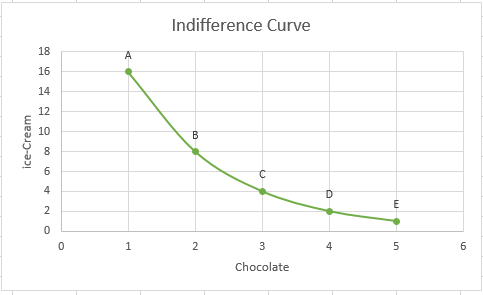In the above graph, points or combinations A, B, C, D, and E provide the same satisfaction level to Nisha. It can also be seen that as Nisha is consuming one additional quantity of chocolate, she has to sacrifice or give up some quantity of ice cream. Therefore, when Nisha moves from Combination A to B to consume one extra chocolate, she has to sacrifice 8 units of ice-creams. Similarly, to move from Combinations B to C, C to D, and D to E, she has to sacrifice 4, 2, and 1 unit of ice-creams, respectively, for the consumption of one extra unit of chocolate at each movement. This sacrifice of units of a good to gain an additional unit of another good is known as the Marginal Rate of Substitution.

Marginal Rate of Substitution can be defined as the amount of Good Y sacrificed to obtain an additional unit of Good X without affecting the total satisfaction level.## Properties of Indifference Curve

#### 1. Indifference Curve always slopes downwards from left to right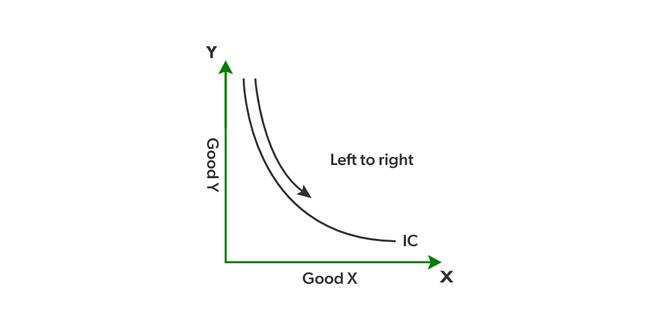An indifference curve is defined as a curve that gives an equal level of satisfaction to a consumer at every possible combination. It is possible when a consumer is willing to sacrifice some quantity of a good to gain an additional unit of another good. If a consumer is having more of a good without any fall in another good, the consumer will achieve a higher satisfaction level instead of equal. This fall in units of one good to gain more of another good gives a downward slope to the indifference curve.

#### 2. Indifference Curves are always convex to the point of origin

The shape of an indifference curve is based on the Diminishing Marginal Rate of Substitution. It means that to gain a single extra unit of a good, a consumer is willing to sacrifice more of another good. As in the case of Nisha (example above), to gain one more unit of chocolate, she is willing to sacrifice more units of ice-creams. This diminishing marginal rate of substitution gives a convex shape to an indifference curve.

However, there are two extreme scenarios for the shape of an indifference curve.

• When two goods are the perfect substitute for each other, the shape of the indifference curve is a straight line. In this case, the Marginal rate of substitution is constant.
• When two goods are perfectly complementary to each other, the shape of the indifference curve is L-shaped and is convex to the origin.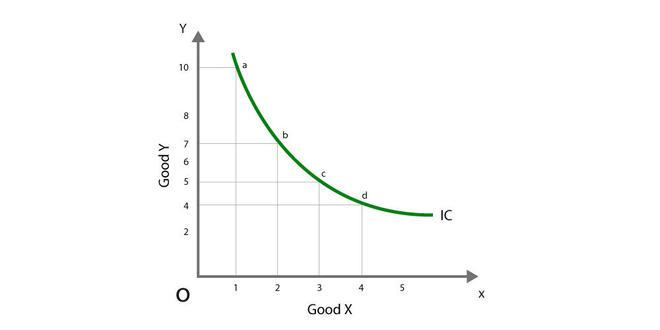As it can be seen in the above image, to attain an additional unit of Good X, i.e., to move from 1 unit to 2 units, the consumer has to sacrifice some units of Good Y, i.e., 3 units (from 10 units to 7 units).

The Diminishing Marginal Rate of Substitution refers to the consumer’s willingness to part with less and less quantity of one good to gain one more additional unit of another good.

#### 3. Higher Indifference Curves represent a higher level of satisfaction

A higher indifference curve represents a higher level of satisfaction, or we can say that an indifference curve to the right of another gives more satisfaction. This property of the indifference curve is based on the assumption of monotonic preference. Monotonic Preference means that a consumer will always prefer a larger bundle, as it gives him/her a higher satisfaction level. In other words, as a consumer prefers more goods, and a higher indifference curve will give a higher satisfaction level.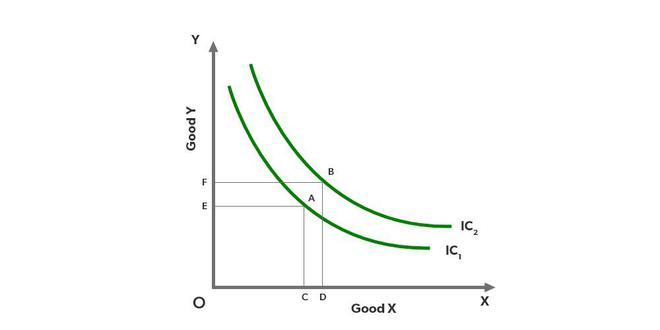After comparing points A and B on IC1 and IC2, respectively, it can be seen that Bundle A involves OC of Good X and OE of Good Y. However, Bundle B involves OD of Good X and OF of Good Y, which shows that the consumer has more goods in Bundle B, which implies more utility or satisfaction level. Therefore, a higher indifference curve means a higher level of satisfaction.

#### 4. Two Indifference Curves cannot intersect each other

An indifference curve consists of different combinations of two goods giving the same satisfaction level to a consumer. It means that every point on an indifference curve gives the same satisfaction to the consumer. Also, an indifference map consists of different indifference curves with different satisfaction levels in each curve. If two indifference curves intersect with each other, it would mean that one point on each curve gives the same level of satisfaction which contradicts the meaning of an indifference map. Therefore, two indifference curves never intersect each other.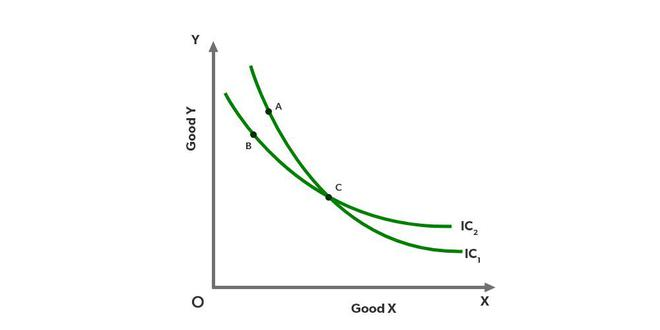If the above figure is true and two indifference curves IC1 and IC2 intersect each other, then it would mean that Point C provides the same satisfaction level to the consumer. However, it has already been proved under Indifference Map that two indifference curves on a single graph show different satisfaction levels along the curve. Therefore, two indifference curves can never intersect each other.

#### 5. An Indifference Curve never touches either of the axes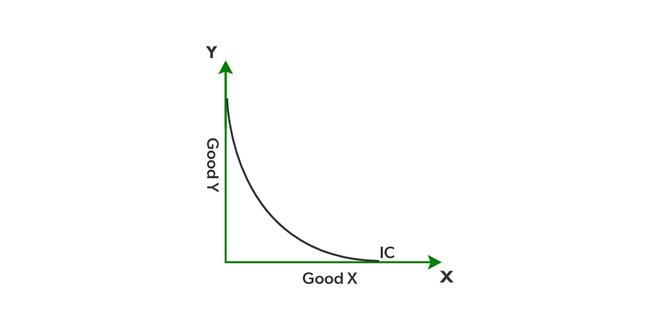The indifference curve is based on the assumption that a consumer considers different possible combinations of two goods and wants both goods. If an indifference curve touches either of the axes, it would mean that a consumer is consuming the whole of one good only, which is not possible and contradicts the assumption. Therefore, an indifference curve never touches either of the axes.

My Personal Notes arrow_drop_up